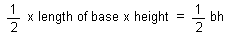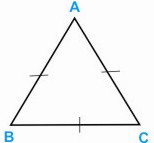HOME MATH DICTIONARY DOWNLOAD FEEDBACK DISCLAIMER
 Question: What is Triangle ? Answer: A triangle is a shape formed from three straight lines, and has three interior angles. It is the simplest polygon. The sum of the interior angles of a triangle is 1800 . p + q + r = 180. The exterior angle of a triangle is equal to the sum of the two opposite interior angles, x = p + r. The area of triangle is given byExample of a Triangle: